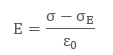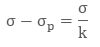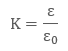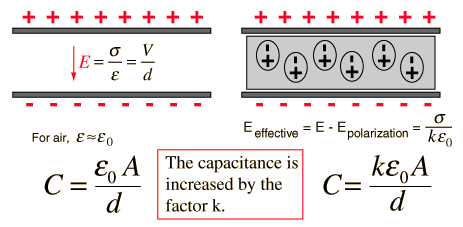> > Effect of Dielectric on Capacitance

# Effect of Dielectric on Capacitance

So far we saw that a bunch of conducting surfaces separated by some space form a device we call a capacitor. Capacitors can hold some quantities of charge. What if a capacitor is put inside a dielectric medium like water or oil? Will the charges leak out or will they stay? Let us find out!

### Suggested VideosElectrostatic ForceElectric field at a point along the axis of Electric DipoleElectrostatic Force## Dielectrics

Dielectrics are basically insulating and non-conducting substances. They are bad conductors of electric current. Dielectrics are capable of holding electrostatic charges while emitting minimal energy. This energy is usually in the form of heat. The common examples of dielectrics include mica, plastics, porcelain, metal oxides and glass etc. It is important for you to note that dry air is also a dielectric.

## What is the Dielectric Constant?

When we put a dielectric slab in between two plates of a parallel plate capacitor, the ratio of the applied electric field strength to the strength of the reduced value of electric field capacitor is called the dielectric constant. It is given as

K = Eo/E

E0 is greater than or equal to E, where Eo is the field with the slab and E is the field without it. The larger the dielectric constant, the more charge can be stored. Completely filling the space between capacitor plates with a dielectric, increases the capacitance by a factor of the dielectric constant:

C = KCo,

where Co is the capacitance with no slab between the plates. This is all about a quick recap. Now let us move ahead and see what effect dielectrics have on the capacitance.

## Effect of Dielectric on Capacitance

We usually place dielectrics between the two plates of parallel plate capacitors. They can fully or partially occupy the region between the plates. When we place the dielectric between the two plates of a parallel plate capacitor, the electric field polarises it.

The surface charge densities are σp and – σp. When we place the dielectric fully between the two plates of a capacitor, then it’s dielectric constant increases from its vacuum value.The electric field inside a capacitor is as follows:Hence we have:Therefore:Ɛ is the permittivity of the substance. The potential difference between the plates is given byFor linear dielectrics:Where k is a dielectric constant of the substance, K = 1.## How does the dielectric increase the capacitance of a capacitor?

The electric field between the plates of parallel plate capacitor is directly proportional to capacitance C of the capacitor. The strength of the electric field is reduced due to the presence of dielectric. If the total charge on the plates is kept constant, then the potential difference is reduced across the capacitor plates. In this way, dielectric increases the capacitance of the capacitor.

##Solved Examples for You

Question:

Assertion: In a circuit where two capacitors with capacitance C1 and C2 are connected in series with C1 followed by C2. A slab is inserted in C2. The potential difference across C2 will decrease.
Reason: The current flows in the clockwise direction, after the introduction of the slab.1. Both the statements are correct and the reason is the correct explanation of the assertion.
2. They both are correct but the reason is not the correct explanation of the assertion.
3. The assertion is correct but the reason is not.
4. The reason is correct but the assertion is not.

Solution: B) Upon inserting the slab, the value of C2 will increase to KC2. In series, V distributes in the inverse ratio of capacitance. As C2 increases, its potential will decrease. As C2 increases, the positive charge on both the capacitors will have to increase. Thus the current will flow in the clockwise direction.

Share with friends

## Customize your course in 30 seconds

##### Which class are you in?
5th
6th
7th
8th
9th
10th
11th
12th
Get ready for all-new Live Classes!
Now learn Live with India's best teachers. Join courses with the best schedule and enjoy fun and interactive classes.Ashhar Firdausi
IIT Roorkee
BiologyDr. Nazma Shaik
VTU
ChemistryGaurav Tiwari
APJAKTU
Physics
Get Started

## Browse

##### Electrostatic Potential and CapacitanceSubscribe
Notify of

## Question Mark?

Have a doubt at 3 am? Our experts are available 24x7. Connect with a tutor instantly and get your concepts cleared in less than 3 steps.